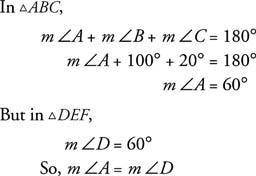## Similar Triangles

In general, to prove that two polygons are similar, you must show that all pairs of corresponding angles are equal and that all ratios of pairs of corresponding sides are equal. In triangles, though, this is not necessary.

Postulate 17 (AA Similarity Postulate): If two angles of one triangle are equal to two angles of another triangle, then the triangles are similar.

Example 1: Use Figure 1 to show that the triangles are similar.Figure 1 Similar triangles.By Postulate 17, the AA Similarity Postulate, Δ ABC ∼ Δ DEF. Additionally, because the triangles are now similar,Example 2: Use Figure 2 to show that ΔQRS ∼ ΔUTS.Figure 2 Similar triangles.

m ∠1 = m ∠2, because vertical angles are equal.

m ∠ R = m ∠ T or m ∠ Q = m ∠ U, because if two parallel lines are cut by a transversal, then the alternate interior angles are equal.

So by the AA Similarity Postulate, Δ QRS∼ Δ UTS.

Example 3: Use Figure 3 to show that Δ MNO∼ Δ PQR.Figure 3 Similar triangles.

In Δ MNOMN = NO, and in Δ PQRPQ = QR.

m ∠ M = m ∠ O and m ∠ P = m ∠ R

(If two sides of a triangle are equal, the angles opposite these sides have equal measures.)

In Δ MNOm ∠ M + m ∠ N + m ∠ O = 180°

In Δ PQRm ∠ P + m ∠ Q + m ∠ R = 180°

Because m ∠ M = m ∠ O and m ∠ P = m ∠ RSo, m ∠ M = m ∠ P, and m ∠ O = m ∠ R. Δ MNO∼ Δ PQR ( AA Similarity Postulate).

Example 4: Use Figure 4 to show that Δ ABC∼ Δ DEF.Figure 4 Similar right triangles.

m ∠ C = m ∠ F (All right angles are equal.)

m ∠ A = m ∠ D (They are indicated as equal in the figure.)

Δ ABC∼ Δ DEF ( AA Similarity Postulate)